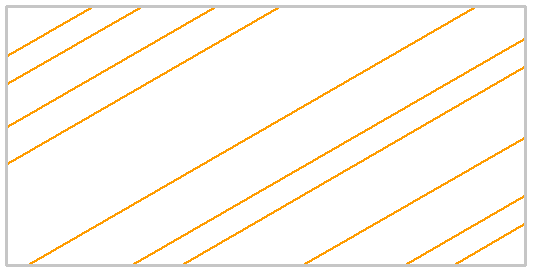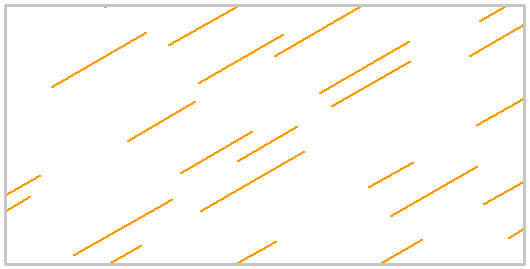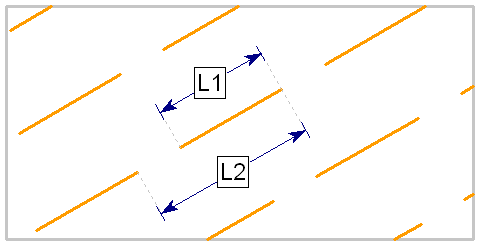# Joint Model: Parallel Statistical

The Parallel Statistical joint network model allows you to define a network of parallel joints with user-defined statistical distributions for the joint spacing, length and persistence.

Parallel Statistical joint network, infinite joint length, random joint spacingParallel Statistical joint network, random joint spacing, length and persistenceThe following input parameters can be defined for the Parallel Statistical joint network model.

## Orientation

The Orientation parameters for the Parallel Statistical joint network model (Trace Plane, Inclination, etc) have the same definition as the Parallel Deterministic model. For complete details, see the Parallel Deterministic topic.

NOTE: for the Parallel Statistical model, the joint orientation CANNOT be assigned a statistical distribution. Since all joints are assumed to be parallel, the joint orientation is a constant value.

## Spacing

The Spacing is the perpendicular distance between the parallel joint planes.

For the Parallel Statistical joint network model, the spacing can be defined as a random variable by selecting a statistical distribution (Normal, Uniform, Exponential or Lognormal), and entering the mean, standard deviation and relative minimum and maximum values. See the Statistical Distributions topic for details about the statistical distribution parameters.

If you do NOT wish to define the spacing as a random variable, then you can choose Distribution = None. In this case, the spacing between all joint planes will be constant and equal to the mean value.

It is important to note that the definition of spacing depends on the setting of the Use Trace Plane option.

• If Use Trace Plane = No, then the spacing is measured in the 2-dimensional cross-sectional plane of the RS2 model (i.e. the apparent spacing, as measured on the trace plane).
• If Use Trace Plane = Yes, then the spacing which you enter must be the actual, 3-dimensional spacing between the joint planes (i.e. the true perpendicular distance between the parallel joint planes, as measured in 3-dimensions).

## Length

For the Parallel Statistical model, the joint length can be specified as infinite, or discontinuous (i.e. user-defined length and persistence), according to the setting of the Infinite Length option.

Infinite Length = Yes

If Infinite Length = Yes, then the joints will extend continuously across the region.

Infinite Length = No

If Infinite Length = No, then you can specify the joint length and persistence, as random variables or as constant values.

To define the Length as a random variable, select a statistical distribution (Normal, Uniform, Exponential or Lognormal), and enter the mean, standard deviation and relative minimum and maximum values. See the Statistical Distributions topic for details about the statistical distribution parameters.

To define the Length as a constant value, choose Distribution = None. In this case, the length of each joint segment will be constant and equal to the mean value.

The Length defines the length of each individual joint in the network, as measured in the plane of the model (i.e. the trace plane). As shown in the figure below, the joint length is the distance L1.

## Persistence

Persistence can only be defined if Infinite Length = No (see above for details).

The Persistence is a measure of joint continuity, and represents the ratio of joint length to total length along the joint planes. The Persistence is a value between 0 and 1. In effect, the Persistence defines the length of intact material between adjacent joint segments. As illustrated in the figure below, the Persistence is equal to the ratio L1/L2.

• To define the Persistence as a random variable, select a statistical distribution (Normal, Uniform, Exponential or Lognormal), and enter the mean, standard deviation and relative minimum and maximum values. See the Statistical Distributions topic for details about the statistical distribution parameters.
• To define the Persistence as a constant value, choose Distribution = None.

For the Parallel Statistical model, if both the joint length and persistence are defined as random variables, then the length of each joint segment, and the gap of intact material between adjacent joint segments, will be variable, according to the statistical distributions you have defined.

The average values of joint spacing, length and persistence for the network should be approximately equal to the mean values, when measured over the entire joint network. This will however depend on the size of the region, relative to the size of joint network (i.e. if only a few joints are generated in the region, then the actual joint spacing, length and persistence will not necessarily match the theoretical distributions you have defined, due to the small sample size).

Definition of joint persistence = L1 / L2## Joint End Condition

For a description of the Joint End Condition option, see the Add Joint Network topic. For further information see the Add Joint Boundary topic.

## Randomize

If you select the Randomize button, the Parallel Statistical joint network will be re-generated, using a new sampling of the random variables (i.e. spacing, length, persistence). The location of the joint network is also randomly generated each time you select Randomize, but the user has no control over the exact location of the joint boundaries, for the Parallel Statistical model.